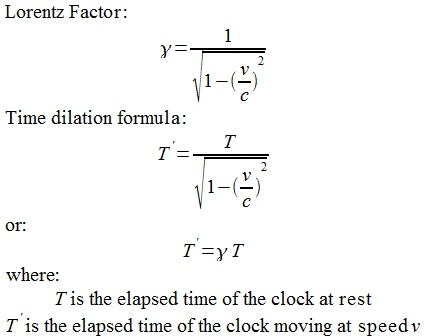What Is Lorentz Factor?

This section introduces Lorentz Factor, which is the factor used in the time dilation and other formulas in special relativity.

What Is Lorentz Factor? Lorentz Factor is the factor used in the time dilation formula that expresses the dilated time of a moving clock observed in a stationary frame. Lorentz Factor also appears in several other formulas in special relativity, see other chapters in this book.

Lorentz Factor is usually written as Greek letter gamma and has value of:

```gamma = 1/sqrt(1-(v/c)**2)   (21) - Lorentz factor
- v is the speed of the moving clock
- c is the speed of light
```

Lorentz Factor has the following properties:

• Lorentz Factor is always greater than 1: gamma > 1.
• Lorentz Factor is very close to 1, if the clock speed, v, is low relative to the speed of light c. For example, the orbiting speed of Earth, v, is about 30,000 m/s, which is about 0.001% of the speed of light c: 300,000,000 m/s. The Lorentz Factor for a clock on the orbiting Earth is about 1.000000005.
• Lorentz Factor become very large, if the clock speed, v, is close to the speed of light c. For example, if a clock is moving at a speed of 99.99% of c, its Lorentz Factor will be 70.71.

If we use Lorentz factor, the time dilation formula can be expressed as:

```T' = (1/sqrt(1-(v/c)**2))*T  (20) - time dilation formula
T' = gamma*T                 (22) - merging (21) into (20)
- Time dilation formula in Lorentz Factor format
```Lorentz Factor and Time Dilation Formula

Last update: 2014.